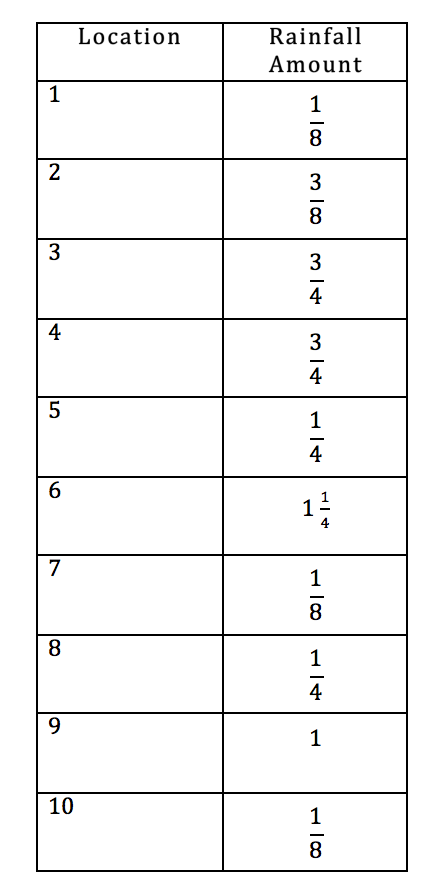# Line Plots & Rainy Days

2 teachers like this lesson
Print Lesson

## Objective

SWBAT create and use a line plot to problem solve.

#### Big Idea

Use science & math skills line plot the amount of rainfall this week.

## Warm-up

10 minutes

As a warm up, students plot 3 given points on a line plot. For the most part, students are successful. Item D was more difficult for some students because they had to convert the improper fraction first.

As students work, I circulate and am able to determine that about 90% of my students "got it". I also use this time to have students read aloud their writing task from yesterday's close to explain how they solved their problem. Students are asked if they can follow others' thinking and drawings/diagrams.

## Guided Practice

20 minutes

A rain gauge is a number line on its side. During guided practice, I explain that we are going to plot the data gathered on a rain gauge from a rainy week. Rather than giving students a sheet, with the rain gauge drawing, I model drawing and labeling a rain gauge. For the first one (Sunday) I have students watch and listen, as I explain and draw.

This is a rain gauge. It's open at the top to collect rain. How do we know how much rain has fallen by looking at a rain gauge? I ask students what the side of the rain gauge reminds them of, and I hold it horizontally so that they can connect it to a number line. I count aloud as I add the unit measures, and then go back and color in 1/2 inch (without announcing the quantity).

What is this rain gauge telling us? Make sure students provide a full response, and don't forget the units! Tell students to copy this rain gauge, and for the time being to not add the "rain" data. We draw and label each rain gauge to practice the increments that are used.

One student was frustrated by the shape, so instead I have her draw and label rectangles. At first, I have the quantity of rain indicated on my model, so that students can determine how much rain "fell" by reading the intervals. Then I switch it up, and give students the fractional amount, and they are to fill in the rain. This last part was a bit more challenging.

Next, we create a line plot. I ask, "What increments should our line plot show?" Students need to make the connection between the gauge increments and the line plot configuration. I have everyone make their own line plot. If you have students struggle with creating a line plot, or those who are such perfectionists that they'll erase and erase you can generate multiple line plots using one of many free online number line generators.

We add in the rain data (yellow), then "match up" fractions that easily add together (marking the off as we add them). One we get our week's total rainfall, we reduce the fraction.

Before I consider this solved, I ask students to justify their work. Then tell them this is the model for what they will be doing during independent practice.

## Independent Practice

20 minutes

A meteorologist set up rain gauges at several spots in Kenansville and recorded the rainfall amounts in the table below. Use the data in the table to create a line plot using 1/8 inch intervals.Some of my students have a very difficult time creating and labeling the line plot, which is the first step in this problem. We have worked on this a lot, and by now with as much practice as we've done, and anchor charts, and technology, I am not sure why students aren't getting this.

So, for the initial work in my independent practice, I guide students in the creation of their line plot and then release them to complete the remaining work. Once they labeled the line plot, they are good to go on their own. They then plot the information, and answer questions based upon the data displayed in the line plot.

## Closure

15 minutes

Students work with their table partner to show/share how they attacked the problem, and their solution. I encourage students to write out a paragraph using a paragraph frame:

My line plot is a number line numbered from ______ to _______. It is broken into _______. Each interval is _________ (don’t forget the unit). ______ is the rain amount that the meteorologist found the most.